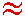Math & Science Home | Proficiency Tests | Mathematical Thinking in Physics | Aeronauts 2000

 CONTENTS Introduction Fermi's Piano Tuner Problem How Old is Old? If the Terrestrial Poles were to Melt... Sunlight Exerts Pressure Falling Eastward What if an Asteroid Hit the Earth Using a Jeep to Estimate the Energy in Gasoline How do Police Radars really work? How "Fast" is the Speed of Light? How Long is a Light Year? How Big is a Trillion? "Seeing" the Earth, Moon, and Sun to Scale Of Stars and Drops of Water If I Were to Build a Model of the Cosmos... A Number Trick Designing a High Altitude Balloon Pressure in the Vicinity of a Lunar Astronaut Space Suit due to Outgassing of Coolant Water Calendar Calculations Telling Time by the Stars - Sidereal Time Fields, an Heuristic Approach The Irrationality ofThe Irrationality ofThe Number (i)i Estimating the Temperature of a Flat Plate in Low Earth Orbit Proving that (p)1/n is Irrational when p is a Prime and n>1 The Transcendentality ofIdeal Gases under Constant Volume, Constant Pressure, Constant Temperature and Adiabatic Conditions Maxwell's Equations: The Vector and Scalar Potentials A Possible Scalar Term Describing Energy Density in the Gravitational Field A Proposed Relativistic, Thermodynamic Four-Vector Motivational Argument for the Expression-eix=cosx+isinx Another Motivational Argument for the Expression-eix=cosx+isinx Calculating the Energy from Sunlight over a 12 hour period Calculating the Energy from Sunlight over actual full day Perfect Numbers-A Case Study Gravitation Inside a Uniform Hollow Sphere Further note on Gravitation Inside a Uniform Hollow Sphere Pythagorean Triples Black Holes and Point Set Topology Additional Notes on Black Holes and Point Set Topology Field Equations and Equations of Motion (General Relativity) The observer in modern physics A Note on the Centrifugal and Coriolis Accelerations as Pseudo Accelerations - PDF File On Expansion of the Universe - PDF File

## Using a Jeep to Estimate the Energy in Gasoline

Problem:
Estimate the energy released in burning a gallon of gasoline in an automobile engine.

Solution:
I used my 1994 Jeep Wrangler to acquire data for this problem. The estimated weight of the Jeep is 3000 pounds, or 1400 kg. On the highway, the jeep travels about 20 miles per gallon of gasoline. The gasoline must be burned to keep the Jeep moving at a constant speed against internal friction and air resistance. To estimate the combined effect of these two forces, I drove until I came to a flat road surface, then shifted into neutral, and allowed the Jeep to decelerate. Counting by thousands to estimate time, it took about 11 sec for the speed to drop from 45 mph to 35 mph. These numbers provide an estimate for the acceleration due to combined friction and air resistance:

(10 mph)/(11 sec) = 0.91 mph/sec = 0.40 m/sec2

and the force slowing the Jeep:

(1400 kg) x (0.40 m/sec2)560 nt

This force is the same force that must be overcome when driving the Jeep at constant speed. Since 20 miles/gal = 32,000 m/gal, the energy in j, expended by burning a gallon of gasoline to overcome 560 nt of force over the distance of one mile, is

(560 nt) x (32,000 m/gal)2 x 107 j/gal.

Since engine efficiency is 20%, the actual energy expended must be about 5 times the above stated amount, or

108 j/gal,

the difference showing up as waste heat. Thus, burning a gallon of gasoline releases about 108 j of energy.

Exploding a ton of TNT liberates about 4 x 109 j, so that a gallon of gasoline is equivalent to about 50 pounds of TNT.